•#### Learning Bayesian Models with R#### Overview of this book

Learning Bayesian Models with RCreditswww.PacktPub.comPrefaceFree Chapter
Introducing the Probability TheoryThe R EnvironmentIntroducing Bayesian InferenceMachine Learning Using Bayesian InferenceBayesian Regression ModelsBayesian Classification ModelsBayesian Models for Unsupervised LearningBayesian Neural NetworksBayesian Modeling at Big Data ScaleIndex## The Naïve Bayes classifier

The name Naïve Bayes comes from the basic assumption in the model that the probability of a particular feature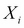is independent of any other feature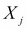given the class label. This implies the following: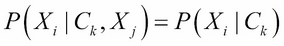Using this assumption and the Bayes rule, one can show that the probability of class, given features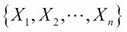, is given by: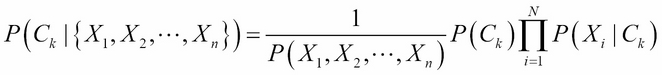Here,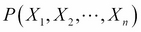is the normalization term obtained by summing the numerator on all the values of k. It is also called Bayesian evidence or partition function Z. The classifier selects a class label as the target class that maximizes the posterior class probability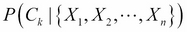: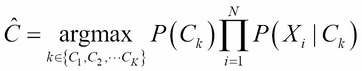The Naïve Bayes classifier is a baseline classifier for document classification. One reason for this is that the underlying assumption that each feature (words or m-grams) is independent of others, given the class label typically holds good for text. Another reason is that the Naïve Bayes classifier scales well when there is a large number of documents.

There are two implementations of Naïve Bayes. In...